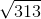## Example Questions

### Example Question #51 : Triangles

2 triangles are similar

Triangle 1 has sides  6, 8, 10

Triangle 2 has sides 5 , 3, x

find x

9

12

5

2

4

4

Explanation:

Draw the triangles

Triangle 1 is a 6,8,10 right triangle with 10 as the hypotenuse

Triangle 2: 3 is half of 6, 5 is half of 10; x must be half of 8

### Example Question #52 : Triangles

Given the diagram, indicate if Quantity A is larger, Quantity B is larger, if they are equal, or if there is not enough information given to determine the relationship.

Quantity A:Quantity B: 7.5

Quantity A is greater.

The relaionship cannot be determined from the information given.

Quantity B is greater.

The two quantities are equal.

Quantity A is greater.

Explanation:

Since this is a 30-60-90 triangle, we know that the length of the side opposite the 60 degree angle is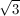times the side opposite the 30 degree angle. Thus,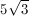, which is about 8.66. This is larger than 7.5.

### Example Question #53 : Triangles

A right triangle's perimeter is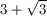. The other two angles of the triangle are 30 degrees and 60 degrees.

Quantity A: The triangle's hypotenuse length

Quantity B: 2

The relationship cannot be determined from the information given.

Quantity B is greater.

Quantity A is greater.

The two quantities are equal.

The two quantities are equal.

Explanation:

The ratio of the sides of a 30-60-90 triangle is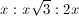, with the hypotenuse being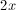. Thus, the perimeter of this triangle would be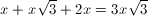. Since the triangle depicted in this problem has a perimeter of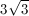must equal 1, which would make the hypotenuse equal to 2.

### Example Question #1 : How To Find The Length Of The Side Of A Right Triangle

If the shortest side of a right triangle has length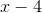and its hypotenuse has length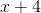, what is the length of the remaining side?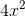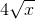Explanation:

Use the Pythagorean theorem,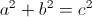, with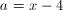and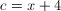, and solve for.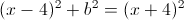Rearrange to isolate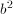: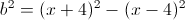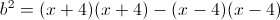Use FOIL to multiply out: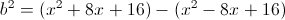Distribute the minus sign to rewrite without parentheses: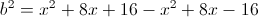Combine like terms: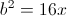Take the square root of both sides: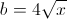### Example Question #3 : How To Find The Length Of The Side Of A Right Triangle

Given the following triangle, what is the length of the unknown side?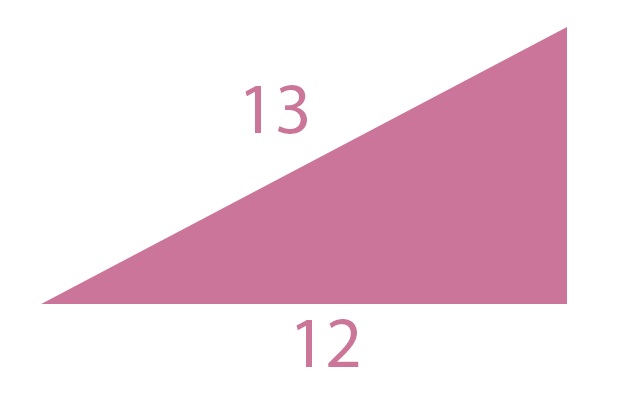The answer cannot be determined from the information given.